# Differential Calculus NAT Level - 2

## 10 Questions MCQ Test Topic wise Tests for IIT JAM Physics | Differential Calculus NAT Level - 2

Description
Attempt Differential Calculus NAT Level - 2 | 10 questions in 45 minutes | Mock test for IIT JAM preparation | Free important questions MCQ to study Topic wise Tests for IIT JAM Physics for IIT JAM Exam | Download free PDF with solutions
*Answer can only contain numeric values
QUESTION: 1

### Maximum area of a rectangle which can be inscribed in a circle of given radius R is given by αR2. Find the value of α.

Solution: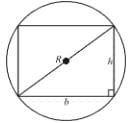Let rectangle has width b and height h.
Area = h·b
Also,  b2 + h2 = (2R)2 = 4R2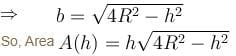Area is maximum when A2 is maxima
A2 = h2(4R2 – h2)]
f(h) = h2(4R2 – h2)
For maxima,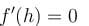⇒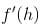= h2(–2h) + (4R2 – h2)2h = 0

h2 + 4R2 – h2 = 0
h2 = 2R2
h = √2

From physical nature of problem, it is clear that this should be maximum area since minimum area will tend towards zero.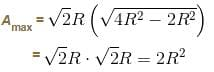Hence, value of α = 2

*Answer can only contain numeric values
QUESTION: 2

### The radius of a right circular cylinder increases at a constant rate. Its altitude is a linear function of the radius and increases three times as fast as radius. When the radius is 1 cm the altitude is 6 cm. When the radius is 6 cm, the volume is increasing at the rate of 1 cm/s. When the radius is 36 cm, the volume is increasing at a rate of n cm3/s. The value of 'n' is equal to :

Solution: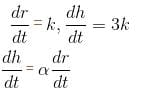h = αr + c
α = 3
h = 3r + c
h = 6, r = 1
c = 3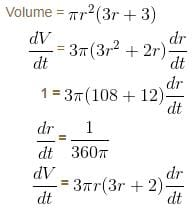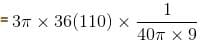= 33 cm3/second

*Answer can only contain numeric values
QUESTION: 3

### The maximum value of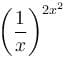is given as (λ/e). The value of  λ is

Solution: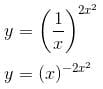⇒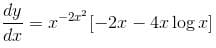For maxima or minima of y.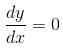⇒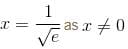and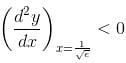Hence, y attains maximum value at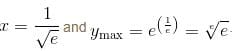The value of λ = 1.

*Answer can only contain numeric values
QUESTION: 4

Consider the function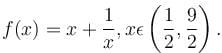If α is the length of interval of decrease and β be the length of interval of increase, then β/α is

Solution: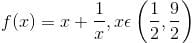f(x) is decreasing in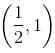and increasing in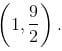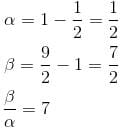*Answer can only contain numeric values
QUESTION: 5

The ratio of absolute maxima and minima of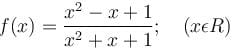is

Solution:

Absolute Maxima = 3
and The Absolute Minima = 1/3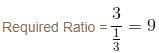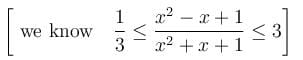*Answer can only contain numeric values
QUESTION: 6

If the interval of monotonicity of the function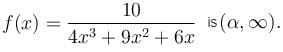Find the value of  α?

Solution: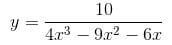⇒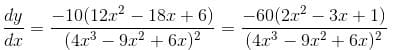⇒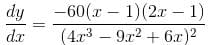⇒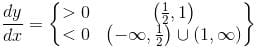Hence,    value of α = 1

*Answer can only contain numeric values
QUESTION: 7

The least area of a circle circumscribing any right triangle of area S is given as απS. Find the value of α.

Solution: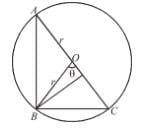Area of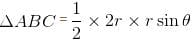S = r2sin θ
⇒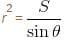Area of circle =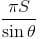Least area = πS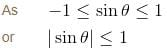So,               Value of α = 1

*Answer can only contain numeric values
QUESTION: 8

Let  f(x) = 2x3 + ax2 + bx - 3cos2 x is an increasing function for all  x∈R  such that  ma2 + nb + 18 < 0 then the value of m + n + 7 is

Solution:

Given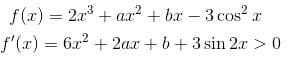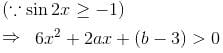D < 0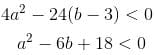m = 1, n = – 6
m + n + 7 = 1 – 6 + 7
= 8 – 6 = 2

*Answer can only contain numeric values
QUESTION: 9

If the maximum value of the function f(x) = (sin-1 x)3 + (cos-1 x)3, -1 << 1 is α and minimum value is β and α - β is of the form n · π3. Find the value of n.

Solution:

Let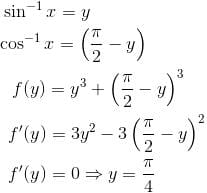π/4  is point of minima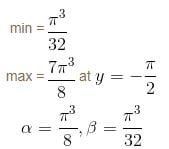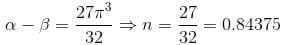*Answer can only contain numeric values
QUESTION: 10

If a, b, c, d are real numbers such that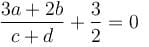then the equation ax3 + bx2 + cx + d = 0  has at least one root in (0, α). Find the value of α.

Solution: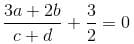⇒  6a + 4b + 3c + 3d = 0
Let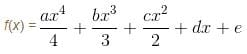f(0) = e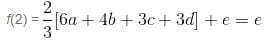Since f(x) is continuous and differentiable in (0,2) and f(0) = f(2) = e

Hence, according to Rolle's Theorem, the equation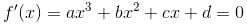has at least one root in (0,2). Thus, value of α = 2.Use Code STAYHOME200 and get INR 200 additional OFF Use Coupon Code